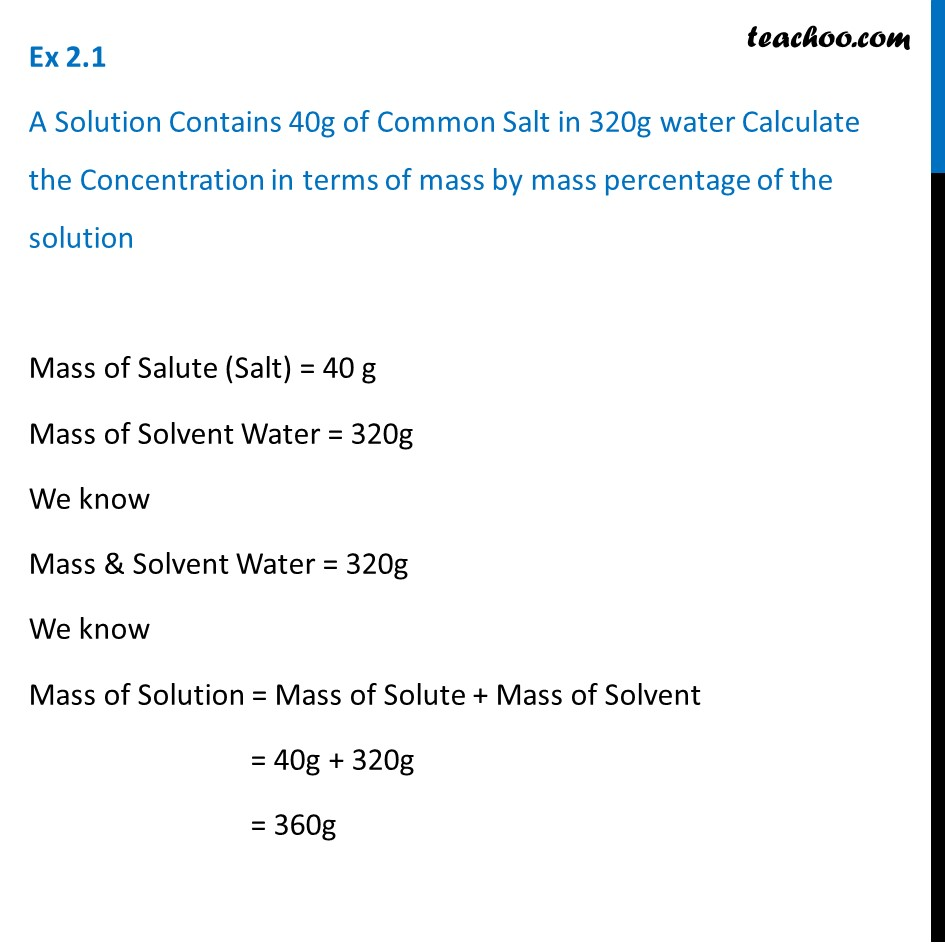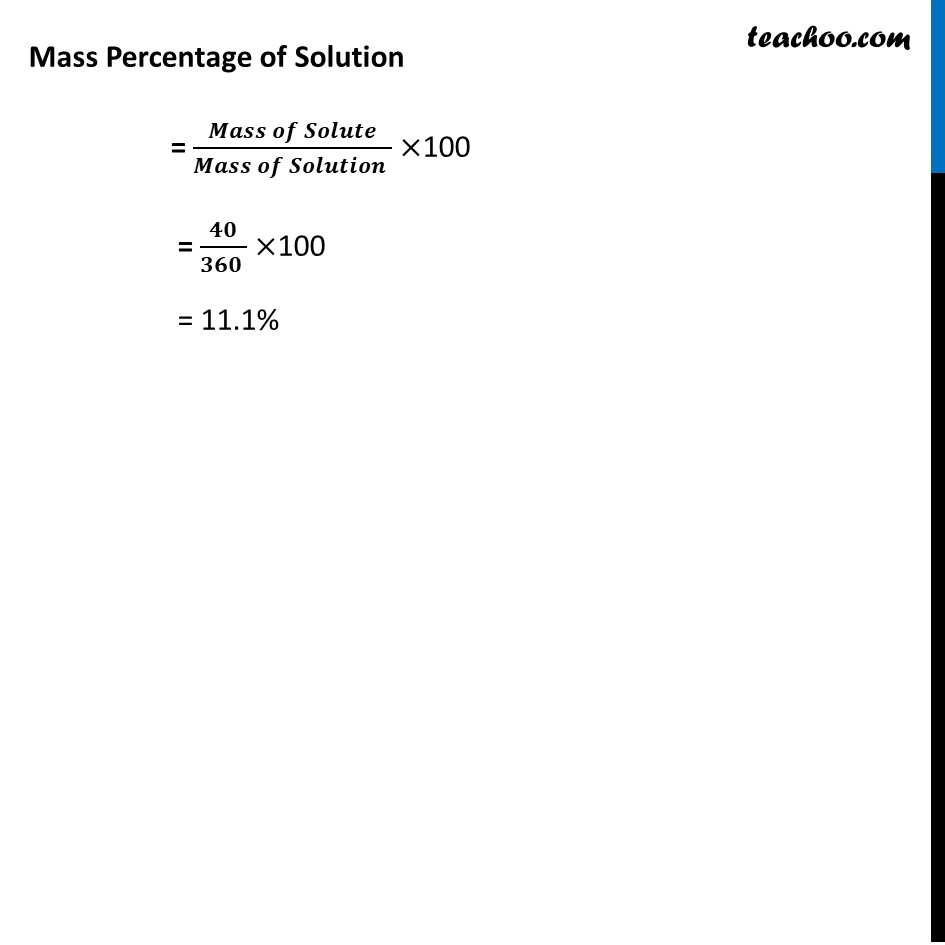Class 9
Chapter 2 Class 9 - Is Matter Around Us PureLearn in your speed, with individual attention - Teachoo Maths 1-on-1 Class

### Transcript

Ex 2.1 A Solution contains 40g of common salt in 320g water calculate the concentration in terms of mass by mass percentage of the solution Mass of salute (Salt) = 40g Mass of Solvent Water = 320 g We know Mass & Solvent Water = 320 g we know Mass of solution = Mass of Solute + Mass of Solvent = 40g + 320g = 360 g Mass Percentage of Solution = mass of solute / mass of solution * 100 = 40/360*100 Mass Percentage of Solution = (𝑴𝒂𝒔𝒔 𝒐𝒇 𝑺𝒐𝒍𝒖𝒕𝒆)/(𝑴𝒂𝒔𝒔 𝒐𝒇 𝑺𝒐𝒍𝒖𝒕𝒊𝒐𝒏 )×100 = 𝟒𝟎/(𝟑𝟔𝟎 )×100 = 11.1%